GMAT Math : DSQ: Calculating the volume of a prism

Example Questions

Example Question #1 : Dsq: Calculating The Volume Of A Prism

Given a right square pyramid and a right cone, which, if either, has greater volume?

Statement 1: The two have the same height.

Statement 2: The base of the cone and the base of the pyramid have the same area.

BOTH statements TOGETHER are sufficient to answer the question, but NEITHER statement ALONE is sufficient to answer the question.

Statement 1 ALONE is sufficient to answer the question, but Statement 2 ALONE is NOT sufficient to answer the question.

Statement 2 ALONE is sufficient to answer the question, but Statement 1 ALONE is NOT sufficient to answer the question.

EITHER statement ALONE is sufficient to answer the question.

BOTH statements TOGETHER are insufficient to answer the question.

BOTH statements TOGETHER are sufficient to answer the question, but NEITHER statement ALONE is sufficient to answer the question.

Explanation:

Given base areaheight, the volume of either a pyramid or a cone is equal to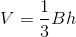.

If we know the heights of the two figures are the same, we still need to know which has the greater base area in order to know which has the greater volume, so Statement 1 is insufficient. By a similar argument, Statement 2 is insufficient.

If we know both heights are equal and that both bases have equal area, then we know that the volumes are equal.

Example Question #2 : Dsq: Calculating The Volume Of A Prism

Find the volume of a rectangular prism.

Statement 1: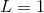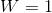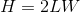Statement 2:  The diagonal is 6.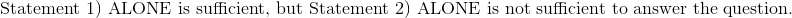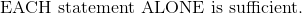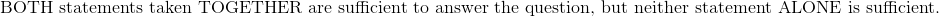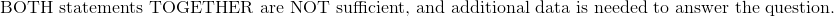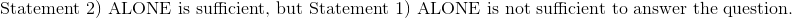Explanation:

Statement 1:The height can be easily solved for by substituting the length and width. Height is 2, and the volume can be calculated by the following formula.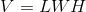Statement 2:  The diagonal is 6.

Write the equation of the diagonal in terms of length, width, and height.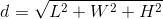Although the lengths from Statement 1 will yield a diagonal of six, there are multiple combinations of length, width, and height which will also give a diagonal of six.  The product of these three dimensions may or may not give a volume of six.  Statement 2 has insufficient information to calculate the volume.

Therefore:Example Question #3 : Dsq: Calculating The Volume Of A Prism

While deep-sea diving, Al found a rectangular treasure chest. Before he opens it, he wants to find the volume of the chest. What is it?

I) Al measured the chest's longest side to be twice its smallest side and one-and-a-half times the length of the medium side.

II) Al found that the chest's shortest side is 2.5ft long.

Either statement is sufficient to answer the question.

Statement I is sufficient to answer the question, but Statement II is not sufficient to answer the question.

Statement II is sufficient to answer the question, but Statement I is not sufficient to answer the question.

Both statements are needed to answer the question.

Both statements are needed to answer the question.

Explanation:

To find the volume of a rectangular solid, use the following formula: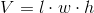Statement I relates the length, width and height of the treasure chest.

Statement II gives us the length of the shortest side of the treasure chest.

Use Statement II and the relationships described in Statement I to find each side, then multiply them all together to get your answer.

If the shortest side is 2.5ft, then the longest side must be 5ft.

If the longest side is 5ft, then the medium side can be found via the following: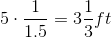So the volume is as follows: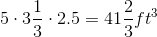Tired of practice problems?

Try live online GMAT prep today.